Our World Statistics Day conversations have been a great reminder of how much statistics can inform our lives. Do you have an example of how statistics has made a difference in your life? Share your story with the Community!
Choose Language Hide Translation Bar
JMP Into R!

This week we celebrate the 35th anniversary of SAS user group meetings with SGF 2010 (formerly known as SUGI). SAS has exhibited extraordinary growth and success since that first meeting of five users in 1975. Over this time span, we have also seen major advancements in the field of modern statistics, especially in the use of computer-intensive methods. One of the leading methods, which coincidently originated around the same time as SAS, is the bootstrap (Efron, 1979). The basic bootstrap method, as this Bootstrapping Page from Wikipedia explains, works by resampling the data with replacement to form an empirical sampling distribution of a statistic of interest. Like SAS, the bootstrap has become a remarkable tool for statistical inference; Efron’s works alone on this method have been cited over 30,000 times.

Fast-forwarding a decade, John Sall (one of the co-founders of SAS) and a small group of developers began working on JMP in 1989. Like many SAS products, JMP continues to evolve and grow in popularity worldwide. Just a few years later, Ross Ihaka & Robert Gentleman began the now widely used open-source statistical language R, with syntax based on the previous S and S+ languages.

SAS Global Forum is an opportunity to learn about how our users employ SAS products, as well as for users to learn about the latest and greatest advancements in upcoming SAS software. One such announcement for JMP 9 (releasing in the fall of 2010) that I am proud to be a part of is the new integration capabilities of JMP with R. I’d like to show the basic elements of the integration in the context of a bootstrap confidence interval simulation example.

JMP is a wonderful complement to R. The integration with R is surfaced with several new JSL commands that allow you to connect to an install of R on your desktop, send data to and from R, and submit R routines available through the R packages. JMP dialogs can easily be built for parameter input to R as a front-end, and more significantly, JMP’s interactive and dynamic statistical platforms and graphics make for a perfect back-end to R functions. The dialog below is an application that connects to R to create data for a given distribution and perform simulations to test the coverage of bootstrap confidence intervals for a few common statistics.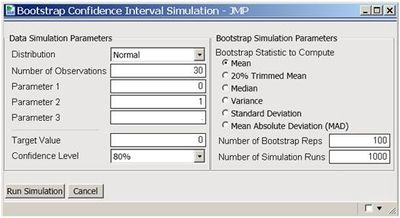You may ask yourself why such a simulation would be interesting or relevant. The bootstrap method (and bootstrap confidence interval) is widely used today to estimate properties of a statistic especially when the distribution of the statistic is not well known. But if that distribution happens to be biased or skewed (such as the distribution of the variance), the bootstrap confidence intervals for the statistic can be inaccurate. This application quickly and easily allows you to evaluate the coverage of bootstrap confidence intervals for the most common distributions and statistics.

The output below shows the results of a simulation (1000 runs) for the 95% confidence intervals of the bootstrapped mean (1000 replications) of a Standard Normal distribution (mean of 0 with variance of 1). The R package “boot” is loaded, and the boot.ci() function computes bootstrap intervals by several different methods. Using the Distribution and Graph Builder platforms in JMP, we see that 95% bootstrap confidence intervals using the Basic, Normal, Percentile, and the BCa method accurately cover the true mean (the Coverage column values equal 1 when the confidence interval contains 0, so the Prob entry for Level 1 corresponds to the empirical coverage). The Graph Builder output shows the confidence interval widths are roughly the same both within and between methods.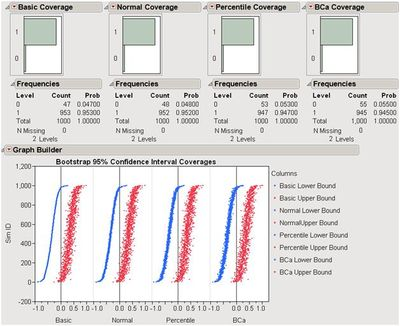Here’s the R integration JSL code used to run the bootstrap for the above scenario:

``````rconn = R Connect();
rconn << Submit("\[
library(boot)

library(boot)

RStatFctn <- function(x,d) {return(mean(x))}

b.basic = matrix(data=NA, nrow=1000, ncol=2)
b.normal = matrix(data=NA, nrow=1000, ncol=2)
b.percent =matrix(data=NA, nrow=1000, ncol=2)
b.bca =matrix(data=NA, nrow=1000, ncol=2)

for(i in 1:1000){
rnormdat = rnorm(30,0,1)
b <- boot(rnormdat, RStatFctn, R = 1000)
b.ci=boot.ci(b, conf =095,type=c("basic","norm","perc","bca")) b.basic[i,] = b.ci\$basic[,4:5]
b.normal[i,] = b.ci\$normal[,2:3]
b.percent[i,] = b.ci\$percent[,4:5]
b.bca[i,] = b.ci\$bca[,4:5]
}
]\"));
b_basic= rconn << Get(b.basic);
b_normal = rconn << Get(b.normal);
b_percent= rconn << Get(b.percent);
b_bca = rconn << Get(b.bca);
rconn << Disconnect();``````

Using the R Connect() JSL command and assigning it to the object “rconn”, the code sends messages to the JSL scriptable object “rconn” to submit R code via the Submit() command and to retrieve R matrices containing the bootstrap confidence intervals back via the Get() commands.

Now let’s see what happens when we test the coverage of bootstrap confidence intervals for the variance of a standard normal distribution. In the dialog, if you change the Target Value to 1 and choose the Variance as the bootstrap statistic to compute and click Run Simulation, you get the results below. In this case, all four methods under-cover the true value of the variance, including the bias-corrected and accelerated (BCa) method proposed specifically to combat bias and skewness in the bootstrapped statistic’s distribution (Efron 1987).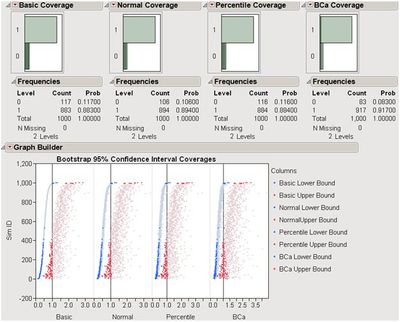Note how easy it is to see the confidence intervals that fail to cover the target value in the Basic bootstrap confidence intervals (by selecting the “0” histogram bar under the Basic Coverage distribution) with new features in JMP 9 that grey out points that were not selected. We can also see how intervals that fail in the Basic method perform in the other methods. Other standard JMP tools such as the Data Filter can help to explore these results in ways that cannot easily and quickly be done in R. Likewise, the comparison of the coverage among methods is easy to see with a custom JSL graphics script to create a Venn diagram that shows the counts of when the intervals contained the true parameter (available on the JMP File Exchange and in JMP Genomics).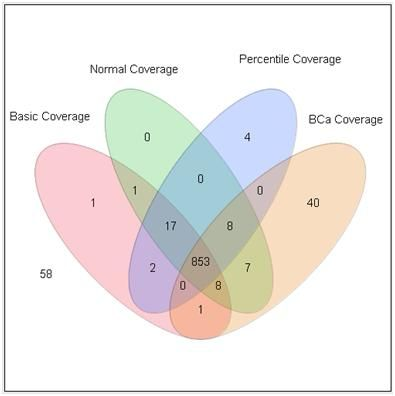This application shows just a taste of what you can do with JMP and R together. With a little JSL and the statistical and graphics platforms of JMP coupled with the breadth and variety of packages and functions in R, one can build complete easy-to-use applications for statistical analysis.

JMP can also integrate with SAS, which adds the ability to work with large-scale data through the file-based system as well as the depth and advanced capabilities of SAS procedures. With these seamless integrations, JMP can become a hub that enables you to connect with both SAS and R, as well as provide unique statistical features such as the JMP Profiler and interactive graphic features such as Graph Builder. The possibilities of what you can do with this are endless!

SAS, JMP, R and the field of statistical computing have come a long way over the last 35 years. I am excited to be a part of this company and this industry as we continue to provide software tools that allow you to accomplish your analyses with flexibility, style, and ease!

When JMP 9 is officially released, I will put the script for the bootstrap simulation and others on the JMP File Exchange.

Now let me leave you with a question: If the bootstrap confidence intervals for the variance of a standard normal distribution are under-covered, how do you think the bootstrap confidence intervals of the mean of a Chi-square distribution will behave?

Article Labels

There are no labels assigned to this post.

Visitor

Ibrahim wrote:

I tried it and that was great, but limited to univariate analysis! Please could you expand or develop it to bivariate and Multivariate platforms? This will enable it's application in a range of tests/analysis. Also the tax bar and help button are not available in the box. I'm using JMP9. Thanks

Visitor

Kelci Miclaus wrote:

Yes! This will be available on the Mac as well when JMP 9 Is released.

Visitor

MS wrote:

Exciting news indeed! I use JMP (Mac OS X) as for hub for most of my data management and analysis needs and R integration would increase that share even further. Please, tell me that this is not to be found in the Windows version only.

Visitor

Giovanni wrote:

I tried using R in JMP and it's working great. I have one issue. I have been trying to change the size of a graphic returned from R into JMP and haven't been able to do it. Is there any parameter or options II can use to change the size of the imported graph. I can change it in R but it will always import it the same size in JMP. Appreciate any help!!

Level IV

I just ran the above script and the JSL Editor flagged an extra right parantheses at Line 19.

Was able to save and run the script afterwards, but with zero output.

No charts, no graphs, nothing.  And the data table is still empty.

What am I doing wrong?  What am I missing?

``````rconn = R Connect();
rconn << Submit("\[
library(boot)
library(boot)
RStatFctn <- function(x,d) {return(mean(x))}
b.basic = matrix(data=NA, nrow=1000, ncol=2)
b.normal = matrix(data=NA, nrow=1000, ncol=2)
b.percent =matrix(data=NA, nrow=1000, ncol=2)
b.bca =matrix(data=NA, nrow=1000, ncol=2)
for(i in 1:1000){
rnormdat = rnorm(30,0,1)
b <- boot(rnormdat, RStatFctn, R = 1000)
b.ci=boot.ci(b, conf =095,type=c("basic","norm","perc","bca")) b.basic[i,] = b.ci\$basic[,4:5]
b.normal[i,] = b.ci\$normal[,2:3]
b.percent[i,] = b.ci\$percent[,4:5]
b.bca[i,] = b.ci\$bca[,4:5]
}
]\");
b_basic= rconn << Get(b.basic);
b_normal = rconn << Get(b.normal);
b_percent= rconn << Get(b.percent);
b_bca = rconn << Get(b.bca);
rconn << Disconnect();``````Community Manager

Are there any errors in the JMP Log window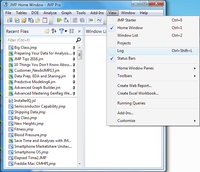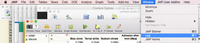Level IV

Jeff,

Log dump below

*:
Interactive HTML: Unsupported display type: LayoutAtomBox
Interactive HTML: Controls are not interactive.
Interactive HTML: Unsupported display type: LayoutAtomBox
Interactive HTML: Internal operation does not support interactive graphs.
Interactive HTML: Embedded profilers in this platform are not interactive.
Interactive HTML: Unsupported display type: LayoutAtomBox
Interactive HTML: Embedded profilers in this platform are not interactive.
Interactive HTML: Unsupported display type: ScrollBox
Interactive HTML: Unsupported display type: ScriptBox
Interactive HTML: Controls are not interactive.
Unexpected ")". Perhaps there is a missing ";" or ",".
Line 19 Column 5: ]\")►);
The remaining text that was ignored was
);b_basic=rconn<<Get(b.basic);b_normal=rconn<<Get(b.normal);b_percent=rconn<<Get
(b.percent);b_bca=rconn<<Get(b.bca);rconn<<Disconnect();
Script as entered:
rconn = R Connect();
rconn << Submit("\[
library(boot)
library(boot)
RStatFctn <- function(x,d) {return(mean(x))}
b.basic = matrix(data=NA, nrow=1000, ncol=2)
b.normal = matrix(data=NA, nrow=1000, ncol=2)
b.percent =matrix(data=NA, nrow=1000, ncol=2)
b.bca =matrix(data=NA, nrow=1000, ncol=2)
for(i in 1:1000){
rnormdat = rnorm(30,0,1)
b <- boot(rnormdat, RStatFctn, R = 1000)
b.ci=boot.ci(b, conf =095,type=c("basic","norm","perc","bca")) b.basic[i,] = b.ci\$basic[,4:5]
b.normal[i,] = b.ci\$normal[,2:3]
b.percent[i,] = b.ci\$percent[,4:5]
b.bca[i,] = b.ci\$bca[,4:5]
}
]\"));
b_basic= rconn << Get(b.basic);
b_normal = rconn << Get(b.normal);
b_percent= rconn << Get(b.percent);
b_bca = rconn << Get(b.bca);
rconn << Disconnect();
Script as parsed:

rconn = R Connect();
rconn << Submit(
"
library(boot)
library(boot)
RStatFctn <- function(x,d) {return(mean(x))}
b.basic = matrix(data=NA, nrow=1000, ncol=2)
b.normal = matrix(data=NA, nrow=1000, ncol=2)
b.percent =matrix(data=NA, nrow=1000, ncol=2)
b.bca =matrix(data=NA, nrow=1000, ncol=2)
for(i in 1:1000){
rnormdat = rnorm(30,0,1)
b <- boot(rnormdat, RStatFctn, R = 1000)
b.ci=boot.ci(b, conf =095,type=c(\!"basic\!",\!"norm\!",\!"perc\!",\!"bca\!")) b.basic[i,] = b.ci\$basic[,4:5]
b.normal[i,] = b.ci\$normal[,2:3]
b.percent[i,] = b.ci\$percent[,4:5]
b.bca[i,] = b.ci\$bca[,4:5]
}
"
);
//:*/
rconn = R Connect();
rconn << Submit("\[
library(boot)
library(boot)
RStatFctn <- function(x,d) {return(mean(x))}
b.basic = matrix(data=NA, nrow=1000, ncol=2)
b.normal = matrix(data=NA, nrow=1000, ncol=2)
b.percent =matrix(data=NA, nrow=1000, ncol=2)
b.bca =matrix(data=NA, nrow=1000, ncol=2)
for(i in 1:1000){
rnormdat = rnorm(30,0,1)
b <- boot(rnormdat, RStatFctn, R = 1000)
b.ci=boot.ci(b, conf =095,type=c("basic","norm","perc","bca")) b.basic[i,] = b.ci\$basic[,4:5]
b.normal[i,] = b.ci\$normal[,2:3]
b.percent[i,] = b.ci\$percent[,4:5]
b.bca[i,] = b.ci\$bca[,4:5]
}
]\"));
b_basic= rconn << Get(b.basic);
b_normal = rconn << Get(b.normal);
b_percent= rconn << Get(b.percent);
b_bca = rconn << Get(b.bca);
rconn << Disconnect();
/*:

Unexpected ")". Perhaps there is a missing ";" or ",".
Line 19 Column 5: ]\")►);
The remaining text that was ignored was
);b_basic=rconn<<Get(b.basic);b_normal=rconn<<Get(b.normal);b_percent=rconn<<Get
(b.percent);b_bca=rconn<<Get(b.bca);rconn<<Disconnect();

TKIntRJMP.R version 5.05
Error: unexpected symbol in:
Error: "b <- boot(rnormdat, RStatFctn, R = 1000)
Error: b.ci=boot.ci(b, conf =095,type=c("basic","norm","perc","bca")) b.basic"

TKIntRJMP.R version 5.05
Error: unexpected symbol in:
Error: "b <- boot(rnormdat, RStatFctn, R = 1000)
Error: b.ci=boot.ci(b, conf =095,type=c("basic","norm","perc","bca")) b.basic"